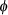A force of 5 pounds stretchesAnonymous

### Question Description

A force of 5 pounds stretches a spring 1 foot. A mass weighing 6.4 pounds is attached to the spring, and the system is then immersed in a medium that offers a damping force numerically equal to 1.6 times the instantaneous velocity.

I calculated that the equation of motion if the mass is initially released from rest from a point 1 foot above the equilibrium position is : x(t) = e^(-4t) [-cos(3t) - 4/3sin(3t)]

Please help me find the equation of motion in the form x(t) = Ae^(??t) sin(sqrt(?^2 - ?^2) +)

-When i tried getting this i got A =4/3, w^2 = 25, lambda = 16, pheta = arctan(3/4)

Find the first time at which the mass passes through the equilibrium position heading upward

_________________S

Please help me find the equation of motion in the form x(t) = Ae^(??t) sin(sqrt(?^2 - ?^2) +)

x(t) = _____________________khakaan (1456)
University of VirginiaReviewAnonymous
Top quality work from this tutor! I’ll be back!Anonymous
It’s my second time using SP and the work has been great back to back :) The one and only resource on the Interwebs for the work that needs to be done!Anonymous
Thanks, good workStudypool4.7Trustpilot4.5Sitejabber4.4Brown University

1271 TutorsCalifornia Institute of Technology

2131 TutorsCarnegie Mellon University

982 TutorsColumbia University

1256 TutorsDartmouth University

2113 TutorsEmory University

2279 TutorsHarvard University

599 TutorsMassachusetts Institute of Technology

2319 TutorsNew York University

1645 TutorsNotre Dam University

1911 TutorsOklahoma University

2122 TutorsPennsylvania State University

932 TutorsPrinceton University

1211 TutorsStanford University

983 TutorsUniversity of California

1282 TutorsOxford University

123 TutorsYale University

2325 Tutors